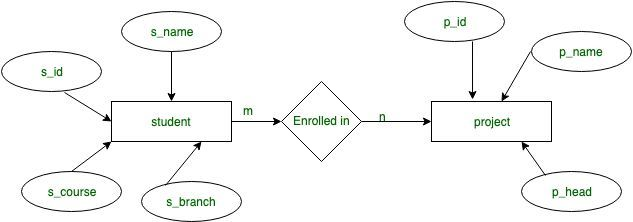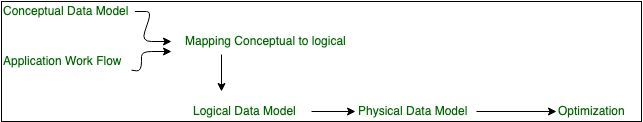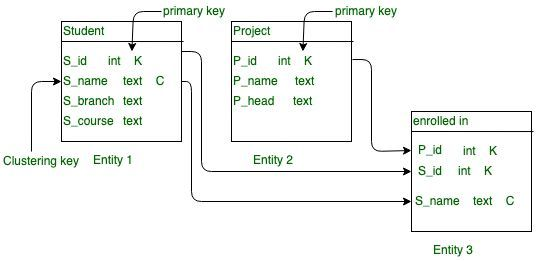# Overview of Data modeling in Apache Cassandra

• Last Updated : 09 Oct, 2019

In this article we will learn about these three data model in Cassandra: Conceptual, Logical, and Physical.

Learning Objective:

• To Build database using quick design techniques in Cassandra.
• To Improve existing model using a query driven methodology in Cassandra.
• To Optimize Existing model via analysis and validation techniques in Cassandra.

Data modelling in Apache Cassandra:
In Apache Cassandra data modelling play a vital role to manage huge amount of data with correct methodology. Methodology is one important aspect in Apache Cassandra. Data modelling describes the strategy in Apache Cassandra.

1. Conceptual Data Model:
Conceptual model is an abstract view of your domain. It is Technology independent. Conceptual model is not specific to any database system.

Purpose:

• To understand data which is applicable for data modeling.
• To define Essential objects.
• To define constraints which is applicable for data modeling.

Advantages of conceptual data modeling in Cassandra is collaboration.

Entity- Relationship(ER) Model:
ER diagram will represent abstract view of data model and give a pictorial view. ER diagram simplified the data model. For example, lets take an example where m:n cardinality in which many to many relationship between student and course means many students can enrolled in many course and many course is enrolled by many students.Figure – ER diagram for conceptual model in Cassandra with M:N cardinality

In this Example s_id, s_name, s_course, s_branch is an attribute of student Entity and p_id, p_name, p_head is an attribute of project Entity and ‘enrolled in’ is a relationship in student record. This is how we will be convert ER diagram into Conceptual data model.

```Student(S_id, S_name, S_branch, S_course)
enrolled in(S_id, P_id, S_name)```

Application Workflow:
In each application there is work flow in which task and dependencies such that In application where number of students want to enroll for many projects.Figure – Data Model Flow diagram

This is actual Data model flow diagram from DataStax.

2. Logical Data Model:
In logical data model we will define each attribute or field or column with functionality such that S_id is a key partition in Student Entity And P_id is a partition key in Project Entity. Partition key is play a vital role in Cassandra in which we can execute query accordingly. In Cassandra Partition key is helpful when we will execute the CQL query and in indexing as well. For example, in Relational database this query would work but in Cassandra it would not work like this.

```Select *
From student_data
Where S_branch = 'CSE'; ```

Because, in Cassandra S_branch is not a part of partition key of the table, so first defined the partition key for such type of Query in Cassandra.

```Select *
From student_data
Where S_id = '123'; ```

This query would work fine in Cassandra.Figure –

3. Physical Data Model:
In this data model we will describe table Query and we will write query to build table and this is one of the actual data model where we need to write Query specifically required and implement the database functionality what we actual want. For example, lets define table one by one for student_record database by using CQL query.

Table: Student

```CREATE TABLE student_record.student
(
S_id int,
S_name text,
S_branch,
S_course,
PRIMARY KEY((S_id), S_name),
);```

Table: Project

```CREATE TABLE student_record.Project
(
P_id int,
P_name text,
PRIMARY KEY(P_id),
); ```

Table: Enrolled_in

```CREATE TABLE student_record.Enrolled_in
(
S_id int,
P_id int,
S_name text,
PRIMARY KEY((S_id, P_id), S_name)),
); ```
My Personal Notes arrow_drop_up
Recommended Articles
Page :